Courses

# Torsion Notes | EduRev

## Mechanical Engineering : Torsion Notes | EduRev

The document Torsion Notes | EduRev is a part of the Mechanical Engineering Course Strength of Materials (SOM).
All you need of Mechanical Engineering at this link: Mechanical Engineering

A shaft is said to be under pure torsion when it is subjected to two equal & opposite couples in a plane perpendicular to the longitudinal axis of the shaft (i.e. twisting couples) in such a way that the magnitude of twisting moment remains constant throughout the length of the shaft.
It’s magnitude is given as the product of the force and the distance between the force.

Torque, T = p x d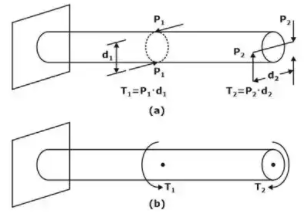Magnitude and representation of Torque

Figure shows a bar or shaft of circular section, subjected to torque T. Such a case is a case of pure torsion,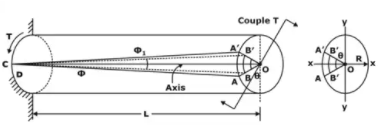Shaft is under pure torsion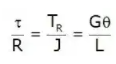J/R is known as torsional section modulus.,& GJ is known as torsional rigidity of the bar or the shaft.
The above relation states that the intensity of shear stress at any point in the cross-section of a shaft subjected to pure torsion is proportional to its distance from the center and the variation of shear stress with respect to radial distance is linear.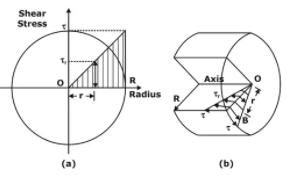Variation of Torsional Shear Stress

Polar moment of inertia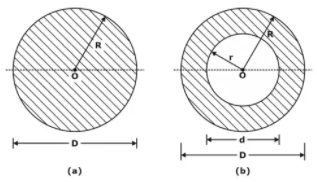(a) For a solid shaft of circular section,
Torsional section modulus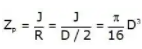(b) For a hollow circular shaft,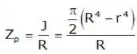Shear Stress Distribution in Different Sections

It is zero at the center and increases in the radially outward direction and become maximum at the outer periphery And for hollow circular shaft, it is minimum at inner radius and maximum at the outer periphery.

• Solid circular section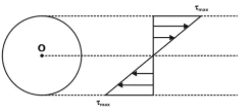• Hollow circular section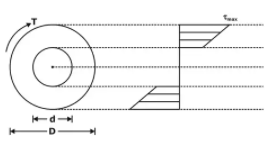Power Transmitted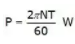Design of Shaft

While designing a shaft, we calculate the maximum torque that can be transmitted from the shaft.
The resisting couple should be equal to the applied torque. Hence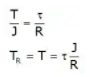Maximum Torque Transmitted by a Circular Shaft
1. Circular Solid Shaft
The maximum torque transmitted by a circular solid shaft is obtained from the maximum shear stress-induced at the outer surface of the solid shaft.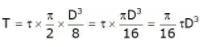2. Hollow Circular Shafts
Torque transmitted by a hollow circular shaft Is obtained in the same way as for a solid shaft,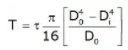Composite Shaft
• Series connection
If two or more shaft of different material, diameter or basic forms are connected together in such a way that each carries the same torque, then the shafts are said to be connected in series & the composite shaft so produced is therefore termed as series connection.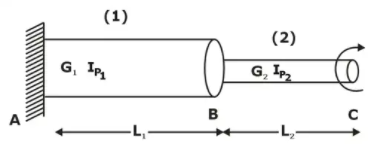for two shafts in series T1 = T2 = T
θAC = θAB + θBC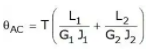• Parallel connection
If two shafts are loaded in such a way that angle of twist on both the shaft is the same then this type of connection is known as a parallel connection of shaft.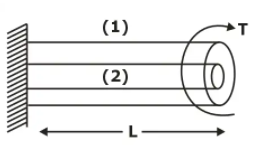For parallel connection of shaft
Torque is cumulative, T = T1 + Tand θ1 = θ2
T1L1 / G1J1 = T2L2 / G2J2

Strain Energy in Torsion

Consider a solid shaft of length L, under the action of torque T.
The torsional strain energy of shaft is equal to the work done in twisting.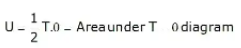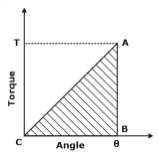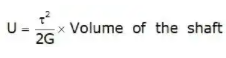Torsional Stiffness (K)

Torsional stiffness is defined as the amount of torque or twisting couple required to produce a twist of unit radian. And it represented by ‘K’
θ = TL / GJ, K = GJ / L

Offer running on EduRev: Apply code STAYHOME200 to get INR 200 off on our premium plan EduRev Infinity!

## Strength of Materials (SOM)

35 videos|33 docs|48 tests

,

,

,

,

,

,

,

,

,

,

,

,

,

,

,

,

,

,

,

,

,

;